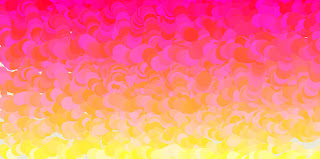### Learning Processing

http://www.processing.org/

Now things start to warm up. Processing looks very promising as a tool to quikly move into interactive realtime graphics. And it's open source. Combining programming wih a graphical output is very satisfying.

Try inserting this code into processing:

void setup(){
size(1024,512,JAVA2D);
x=0;
y=height/2;
colourR=random(0,255);
colourG=random(0,255);
colourB=random(0,255);
speed=5;
}

void draw() {
x=x+speed+random(-2,2);
y=y+(sin(x/15)*random(2,20));
noStroke();
fill((255),(y/2),random(50,200));
smooth();
void mouseReleased() { x=mouseX;
y=mouseY;
speed=random(2,20);
}

This results in a generated image like this: